Study Guide

# Proving Triangle Similarity

## Proving Triangle Similarity

Imagine you've been caught up in a twister that deposits you and your little dog in the middle of a strange new land. In order to get home, you must prove that two triangles are similar. How will you do it?

Just click your heels together three times and say, "There's no place like my geometry classroom. There's no place like my geometry classroom. There's no place like my geometry classroom."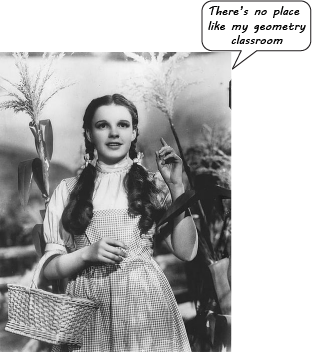If only!

Back here in the real world, there are three methods we can use to prove that two triangles are similar. Fortunately, they're pretty similar to a few we've already learned about. See what we did there? Aren't we a hoot?

The first time we saw these methods, they looked like helpful farmhands, tending the congruent pigs and plowing the congruent fields. Now, they're a scarecrow, a tin man, and a lion, and they're all about helping you get to the similarity wizard at the end of the Yellow Brick Road. They may look like the same theorems (in some cases, they even share the same names), but they're not the same. Those other ones were about congruent triangles, and these ones are about similar triangles.

The first method of proving similarity is the Side-Side-Side (SSS) Postulate. Here's what it says about similar triangles: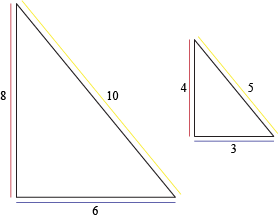If the three sides of the two triangles are proportional in length, then the triangles are similar.

### Sample Problem

Prove that these triangles are similar.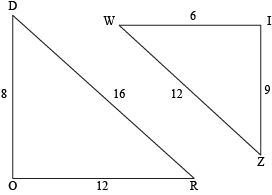Statement Reason 1. DO = 8, WI = 6 Given 2. OR = 12, IZ = 9 Given 3. RD = 16, ZW = 12 Given 4.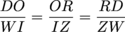Algebra 5. ∆DOR ~ ∆WIZ Side-Side-Side Postulate (4)

The second way to prove triangle similarity is the Angle-Angle (AA) Postulate. It goes something like this: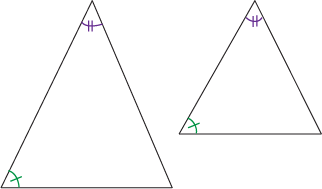If two triangles have two pairs of congruent angles, then the triangles are similar.

### Sample Problem

Prove that these triangles are similar.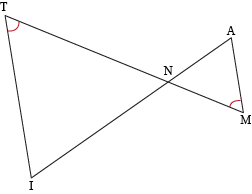Statement Reason 1. ∠T ≅ ∠M Given (in figure) 2. ∠TNI ≅ ∠MNA Vertical Angles Theorem 3. ∆TIN ~ ∆MAN Angle-Angle Postulate (1, 2)

There's one more way to prove that two triangles are similar: the Side-Angle-Side (SAS) Postulate. SAS is a nice little mash-up of AA and SSS. Kind of the way that flying monkeys are mash-ups of birds and monkeys, except the SAS is a lot more civilized and doesn't take its orders from a water-soluble witch. We think.

Basically, what it says is this: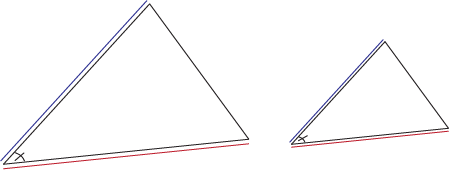If two side lengths are proportional and the angle in between those two sides is congruent, then the triangles are similar.

### Sample Problem

Prove that these triangles are similar.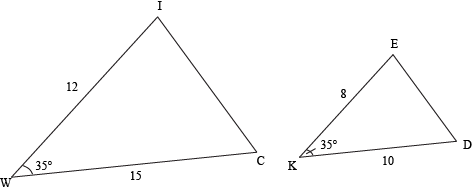Statement Reason 1. WI = 12, WC = 15, KE = 8, KD = 10 Given 2.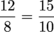Algebra 3.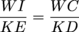Substitution Property 4. m∠W = 35, m∠K = 35 Given 5. ∠W ≅ ∠K Transitive Property 6. ∆WIC ~ ∆KED Side-Angle-Side (3, 5)